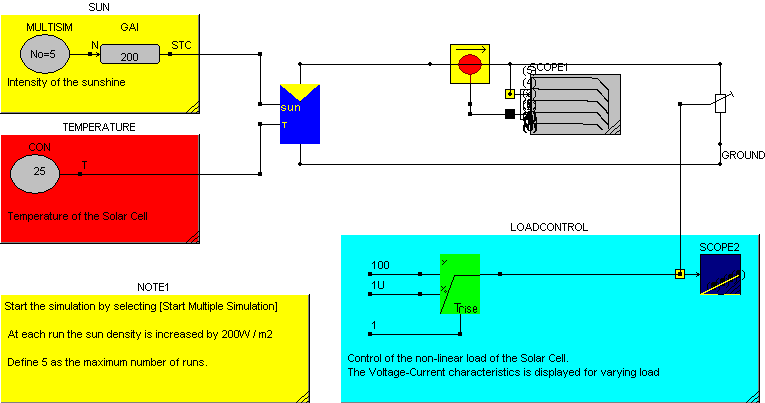• Introduction
• What is in this manual
• What is Caspoc
• User interface
• Introduction
• Starting
• Simulation
• Editing
• Viewing and printing
• Getting Started
• Basic editing
• Simulation in the time domain
• Basic User Interface Topics
• Editing
• Simulation
• Viewing
• Library
• Reports
• Project management
• Circuit and Block Diagram Components
• Introduction
• Cscript and user defined functions
• Component parameters
• Modeling Topics
• Introduction
• Power Electronics
• Semiconductors
• Electrical Machines
• Electrical drives
• Power Systems
• Mechanical Systems
• Thermal Systems
• Magnetic Circuits
• Green Energy
• Coupling to FEM
• Experimenter
• Analog hardware description language
• Embedded C code Export
• Coupling to Spice
• Small Signal Analysis
• Matlab coupling
• Tips and tricks
• Appendices

## Solar Cell

Modeling a solar Cell based on the Voltage/Current characteristic.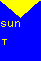The model is based on the Voltage/Current characteristic provided by the manufacturer of the Solar Cell.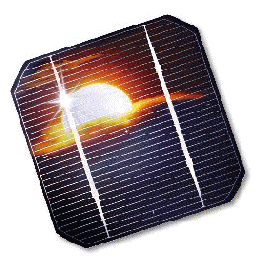The word Photo-voltaic is a combination of the Greek word for Light and the name of the physicist Allesandro Volta. It identifies the direct conversion of sunlight into energy by means of solar cells. The conversion process is based on the photoelectric effect discovered by Alexander Bequerel in 1839. The photoelectric effect describes the release of positive and negative charge carriers in a solid state when light strikes its surface.

How Does a Solar Cell Work?

Solar cells are composed of various semi-conductive materials. Semiconductors are materials, which become electrically conductive when supplied with light or heat, but which operate as insulators at low temperatures. Over 95% of all the solar cells produced worldwide are composed of the semiconductor material Silicon (Si). As the second most abundant element in earth's crust, silicon has the advantage, of being available in sufficient quantities, and additionally processing the material does not burden the environment. To produce a solar cell, the semiconductor is contaminated or "doped". "Doping" is the intentional introduction of chemical elements, with which one can obtain a surplus of either positive charge carriers (p-conducting semiconductor layer) or negative charge carriers (n-conducting semiconductor layer) from the semiconductor material. If two differently contaminated semiconductor layers are combined, then a so-called p-n-junction results on the boundary of the layers.

At this junction, an interior electric field is built up which leads to the separation of the charge carriers that are released by light. Through metal contacts, an electric charge can be tapped. If the outer circuit is closed, meaning a consumer is connected, then direct current flows.

Silicon cells are approximately 10 cm by 10 cm large (recently also 15 cm by 15 cm). A transparent anti-reflection film protects the cell and decreases reflective loss on the cell surface.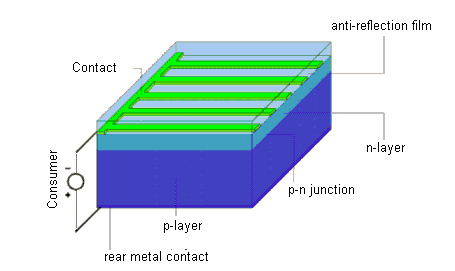Characteristics of a Solar Cell

The usable voltage from solar cells depends on the semiconductor material. In silicon it amounts to approximately 0.5 V. Terminal voltage is only weakly dependent on light radiation, while the current intensity increases with higher luminosity. A 100 cm� silicon cell, for example, reaches a maximum current intensity of approximately 2 A when radiated by 1000 W/m�.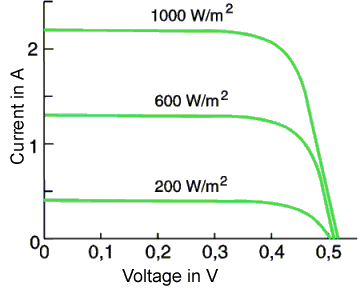The output (product of electricity and voltage) of a solar cell is temperature dependent. Higher cell temperatures lead to lower output, and hence to lower efficiency. The level of efficiency indicates how much of the radiated quantity of light is converted into useable electrical energy.

From the Cell to the Module

In order to make the appropriate voltages and outputs available for different applications, single solar cells are interconnected to form larger units. Cells connected in series have a higher voltage, while those connected in parallel produce more electric current. The interconnected solar cells are usually embedded in transparent Ethyl-Vinyl-Acetate, fitted with an aluminum or stainless steel frame and covered with transparent glass on the front side.

The typical power ratings of such solar modules are between 10 Wpeak and 100 Wpeak. The characteristic data refer to the standard test conditions of 1000 W/m� solar radiation at a cell temperature of 25� Celsius. The manufacturer's standard warranty of ten or more years is quite long and shows the high quality standards and life expectancy of today's products.

To understand the parameters of a solar cell, the parameters for a Shell SP150 Solar Module are derived. This cell has 72 Solar cells in series and each cell is 125mm * 125 mm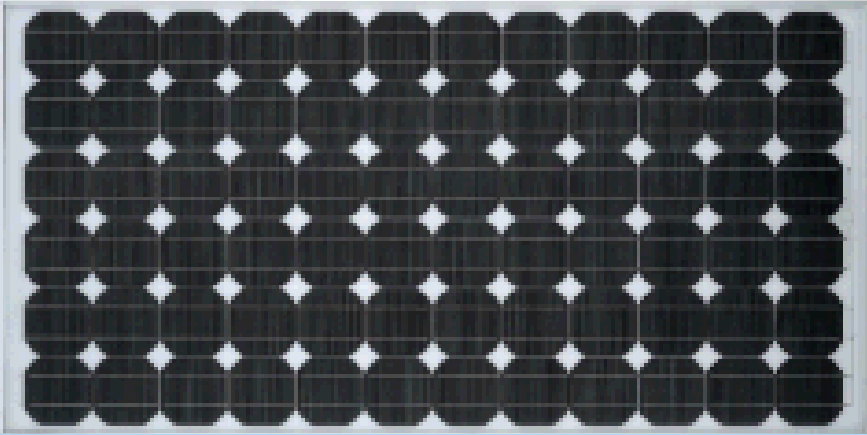The electrical parameters for the Shell module are taken from the data sheet:

• Nominal Voltage 24 [V]
• Maximum Power Point MPP 150[W]
• Rated current IMPP 4.4 [A]
• Rated voltage VMPP 34.0 [V]
• Short circuit current ISC 4.8 [A]
• Open circuit voltage VOC 43.4 [V]

The characteristics are dependent on the sunlight. The maximum current is proportional to the sunlight density. The density of the sunlight is defined in W/m2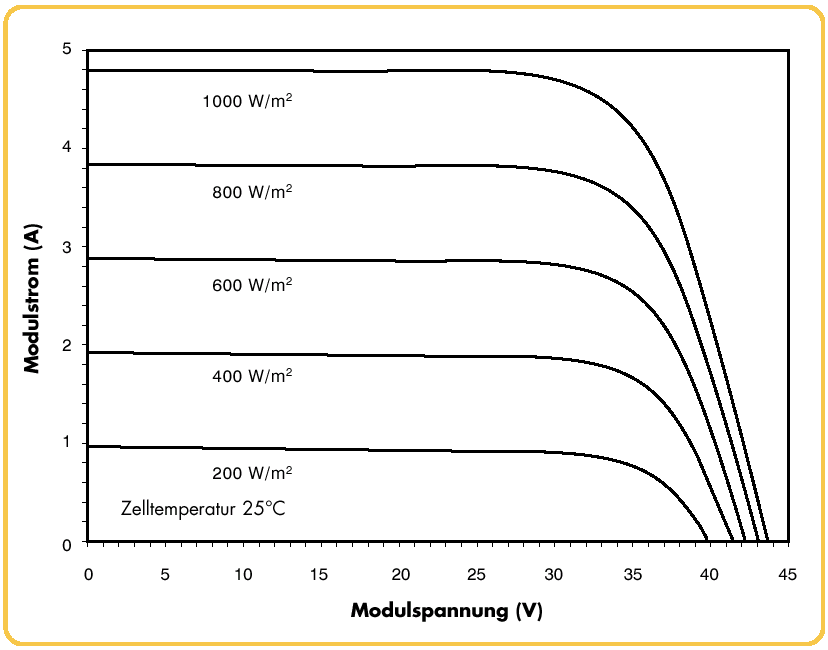When the temperature is rising, the open circuit voltage VOC(i=0) is getting lower.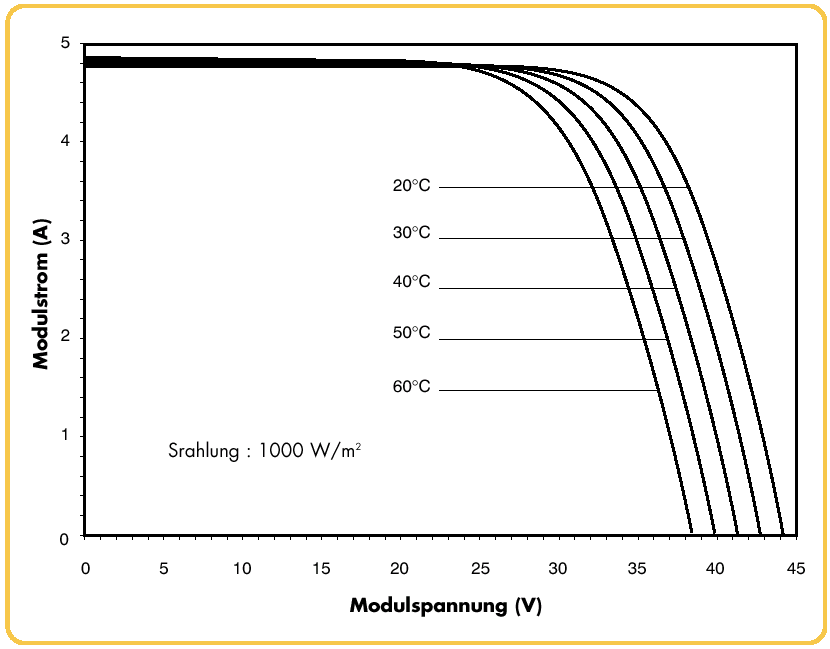The thermal parameters are given in the datasheet:

• Temperature coefficient of the short-circuit current ISC +2.06 mA / �C
• Temperature coefficient of the open-circuit voltage VOC -0.152 V / �C

Select the solar block from the library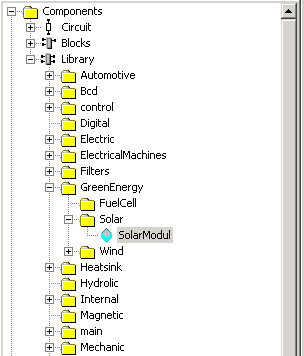The inputs on the left side are the sunlight density and the temperature. The terminals on the top and bottom are the electrical terminals.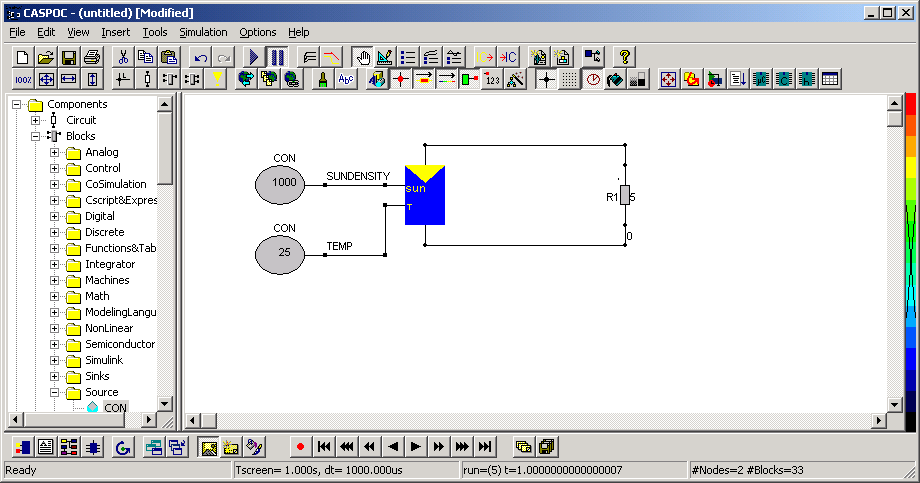The solar module properties dialog box is opened by licking hte block with the right mouse button.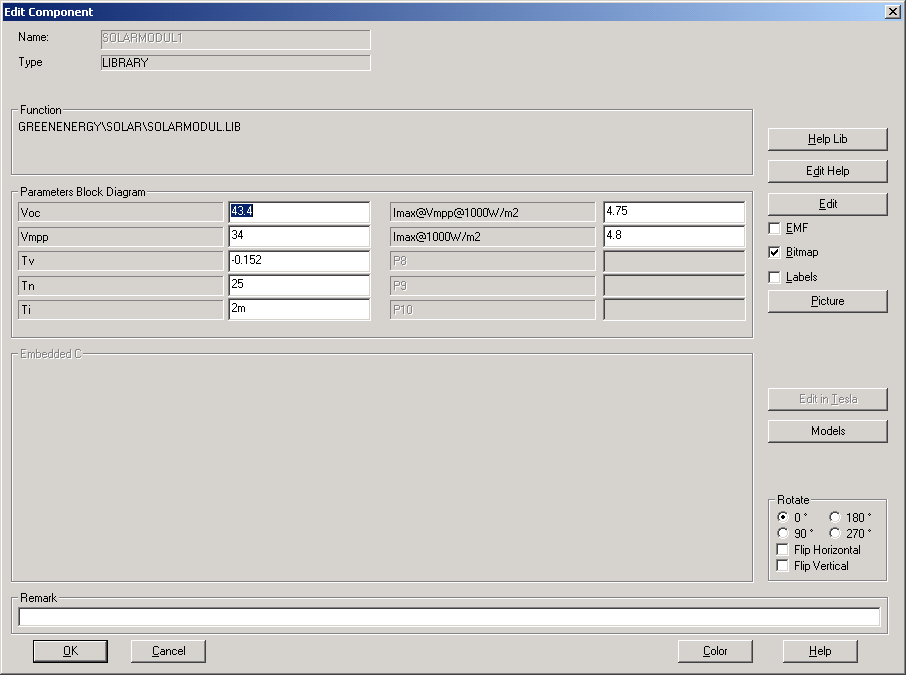In this properties dialog box, the parameters are specified that model the sunlight and temperature dependency of the solar cell.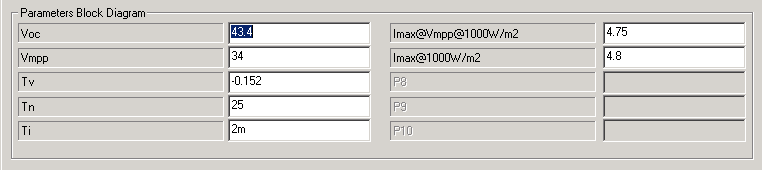The parameters are defined for a sunlight density of 1000W/m2 and a temperature of 25�C

• Open circuit voltage VOC=43.4[V] is the voltage of cell when there is no current drawn from the cell.
• Maximum Power Point voltage VMPP=34.0[V] is the voltage at which the cell delivers the maximum power.
• Temperature coefficient Tv=-0.152 V/�C of the open-circuit voltage VOC
• Nominal Temperature Tn=25�C
• Temperature coefficient Ti=+2.06 mA/�C of the short-circuit current ISC
• Maximum current Imax@VMPP@1000W/m2=4.75[A] is the maximum current at the Maximum Power Point.
• Maximum current Imax@1000W/m2=4.8[A] is the maximum current at short circuit.

If the resistive load ofthe solar cell equals 5 Ohm, the cross-section of the load characteristic R=V/I with the solar cell characteristic shows that the solar cell will produce 24 volts for a current of nearly 4.8 Ampere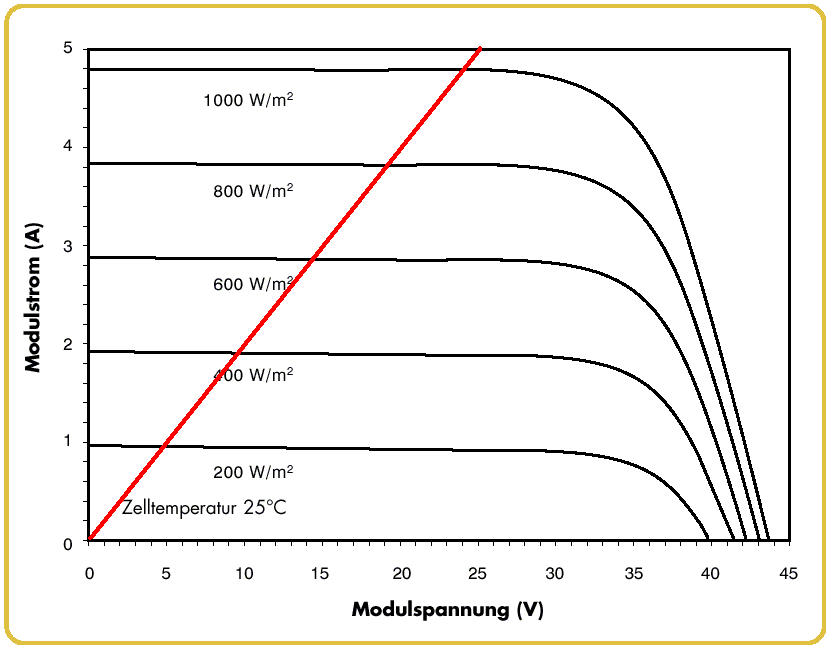If the simulation is started we can see that the voltage over the resistor equals 23.824Volt and the current through the resistor equals 4.765 Ampere. (The values are displayed at the bottom-right corner of the window, if hte mouse os moved over the component.)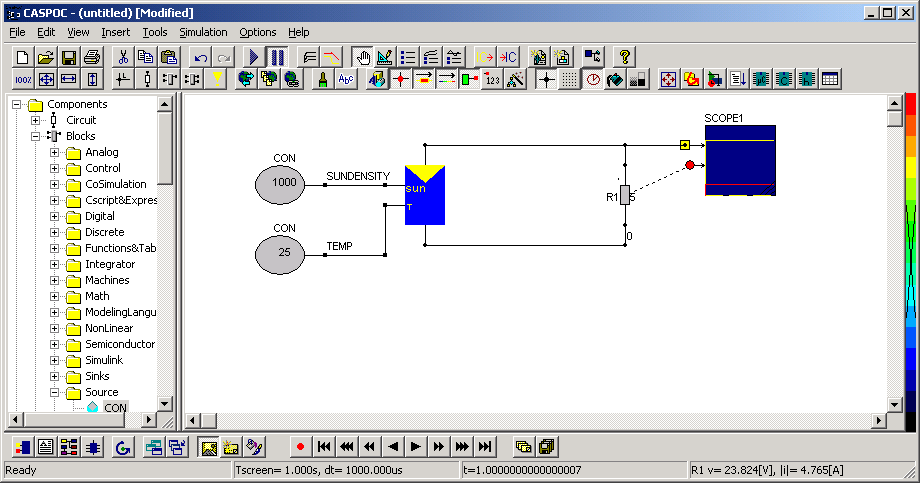The characteristics for the solar module can be simulated if the sunlight density and temperature are varied, as it is done in the simulation below.